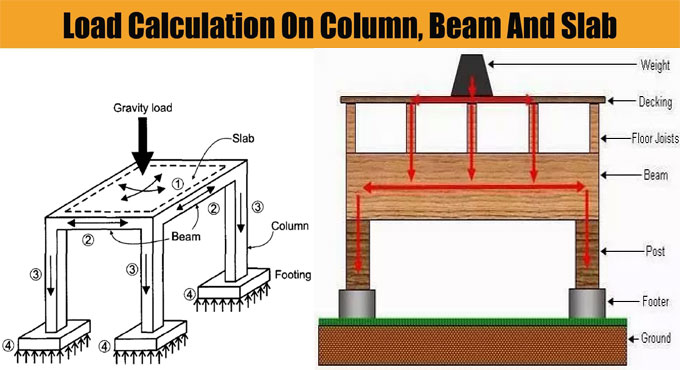# Some useful tips to measure loads on column, beam and slabIn order to work out the total load on columns, Beam and Slab, there should be clear ideas on the types of loads enforcing on the column.

1) Column Self Weight X Number of floors
2) Beams Self Weight per running meter
3) Load of walls per running meter

Apart from above loading, the columns are also susceptible to bending moments which should be taken into consideration in the final design.

For Colomn: The Self weight of Concrete remains approx 2400 kg/m3, that is similar to 240 kN and self weight of steel is approx 8000 kg/m3.

Therefore, if we consider a column size of 230 mm x 600 mm with 1% steel and 3 meters standard height, the self weight of column is approx 1000 kg per floor that is equivalent to 10 kN.

At the time of making calculation, self weight of columns is taken as 10 to 15 kN per floor.

For Beam: Similar method is also used for making calculations of beam. Suppose, each meter of beam contains dimensions of 230 mm x 450 mm without slab thickness. Therefore, the self weight should be approx 2.5 kN per running meter.

For Walls: The Density of bricks differs among 1500 to 2000 kg per cubic meter. For a brick wall with thickness 6 inch, height 3 meter a length 1 meter. The load / running meter should be equivalent to 0.150 x 1 x 3 x 2000 = 900 kg, that is identical to 9 kN/meter. This method is useful for working out the load of brick per running meter for any brick type.

For aerated concrete blocks and autoclaved concrete blocks similar to Aerocon or Siporex, the weight per cubic meter should remain 550 to 700 kg per cubic meter.

When these blocks are utilized for construction, the wall loads for each running meter should remain as low as 4 kN/meter, the cost of the project is decreased considerably with the use of this block.

For Slab: Suppose, the slab contains thickness of 125 mm.

Therefore, self weight of each square meter of slab should be = 0.125 x 1 x 2400 = 300 kg that is identical to 3 kN.

Now, If finishing load is taken to be 1 kN per meter and superimposed live load to be 2 kN per meter. Therefore, from above data, the load of slab can be calculated as 6 to 7 kN approximately per square meter.

Factor of Safety: At the end, once the total load on a column is computed, consider the factor of safety that is very crucial for any building design for safe and convenient performance of building during its design life cycle.